# Class 12 Maths NCERT Solutions for Chapter 8 Application of Integrals Miscellaneous Exercise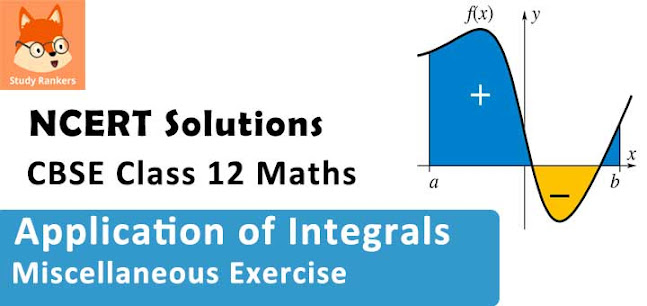### Integrals Miscellaneous Exercise Solutions

1. Find the area under the given curves and given lines:
(i) y = x2x = 1, x = 2 and x-axis
(ii) y = x4x = 1, x = 5 and x –axis

Solution

(i) The required area is represented by the shaded area ADCBA as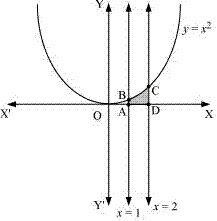Area ADCBA =  ∫12 y dx
= ∫12 x2 dx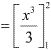= 8/3 - 1/3
= 7/3 units

(ii) The required area is represented by the shaded area ADCBA as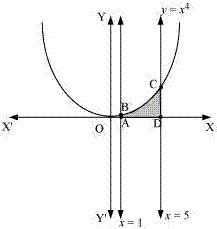Area ADCBA = ∫15 x4 dx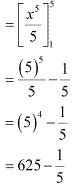= 624.8 units

2. Find the area between the curves y = x and y = x2.

Solution

The required area is represented by the shaded area OBAO as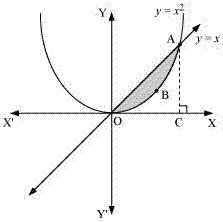The points of intersection of the curves, y = x and y = x2, is A (1, 1)
We draw AC perpendicular to x - axis.
Area (OBAO) = Area (ΔOCA) - Area (OCABO) ...(1)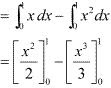= 1/2 - 1/3
= 1/6 units

3. Find the area of the region lying in the first quadrant and bounded by y = 4x2x = 0, y = 1 and = 4.
Solution
The area in the first quadrant bounded by y = 4x2 , x = 0, y = 1 and y = 4 is represented by the shaded area ABCDA as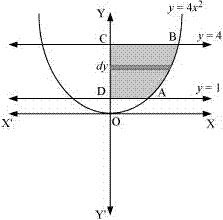Area ADCBA =  ∫14 y dx
= ∫14 √y/2  dx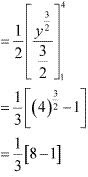= 7/3 units

4. Sketch the graph of y = |x + 3| and evaluate ∫-60 |x + 3| dx.
Solution
The given equation is y = |x + 3|
The corresponding values of x and y are given in the following table :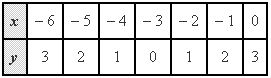On plotting these points, we obtain the graph of y = |x + 3| as follows.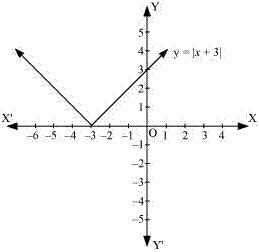It is known that, (x + 3) ≤ 0 for -6 ≤ x ≤ -3 and (x + 3) ≥ 0 for -3 ≤ x ≤ 0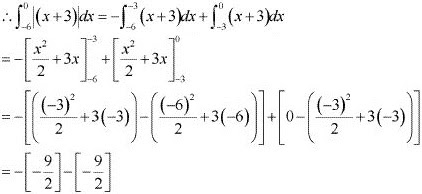= 9

5. Find the area bounded by the curve y = sin between x = 0 and x = 2π.
Solution
The graph of y = sin x can be drawn as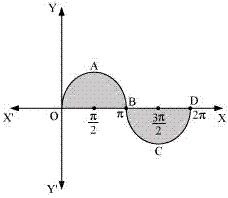∴ Required area = Area OABO + Area BCDB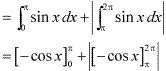= [ - cosπ + cos 0] + |- cos 2π + cos π|
= 1 + 1+ |(-1-1)|
= 2 + |-2|
= 2 + 2 = 4 units

6. Find the area enclosed between the parabola y2 = 4ax and the line y mx.
Solution
The area enclosed between the parabola, y2 = 4ax and the line y mx, is represented by the shaded area OABO as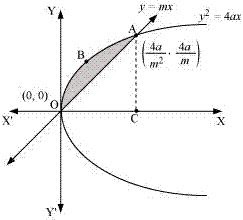The points of intersection of both the curves are (0, 0) and (4a/m2, 4a/m)
We draw AC perpendicular to x - axis.
Area OABO = Area OCABO - Area (ΔOCA)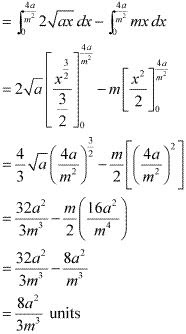7. Find the area enclosed by the parabola 4y = 3x2 and the line 2y = 3x + 12
Solution
The area enclosed between the parabola, 4y = 3x2 , and the line, 2y = 3x + 12, is represented by the shaded area OBAO as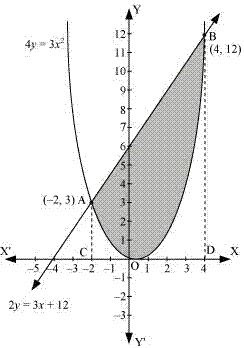The points of intersection of the given curves are A(-2, 3) and (4, 12).
We draw AC and BD perpendicular to x - axis.
Area OBAO = Area CDBA - (Area ODBO + Area OACO)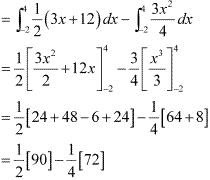= 45 - 18
= 27 units

8. Find the area of the smaller region bounded by the ellipse x2 /9 + y2 /4 and the line x/3 + y/2 = 1.
Solution
The area of the smaller region bounded by the ellipse, x2/9 + y2/4 = 1, and the line x/3 + y/2 = 1 , is represented by the shaded region BCAB as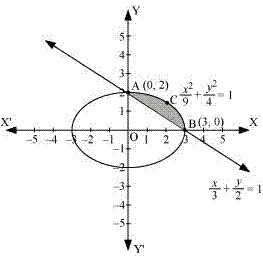Area BCAB = Area (OBCAO) - Area (OBAO)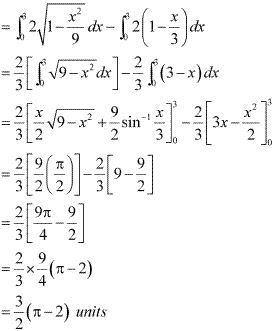9. Find the area of the smaller region bounded by the ellipse x2 /a2  + y2 /b2  = 1 and the line x/a + y/b = 1.
Solution
The area of the smaller region bounded by the ellipse, x2 /a2  + y2 /b2  = 1 and the line, x/a + y/b = 1, is represented by the the shaded region BCAB as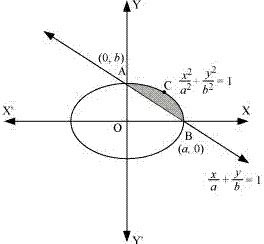Area BCAB = Area (OBCAO) - Area (OBAO)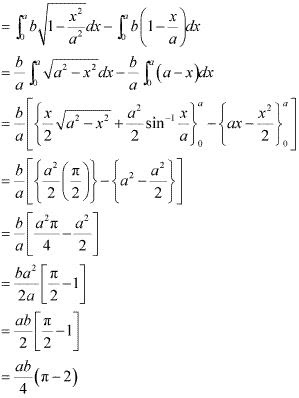10. Find the area of the region enclosed by the parabola x2 = y, the line y = x + 2 and x-axis.
Solution
The area of the region enclosed by the parabola, x2 = y , the line, y = x + 2 and x - axis is represented by the shaded region OABCO as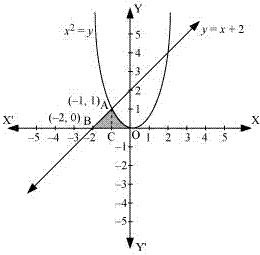The point of intersection of the parabola, x2 = y, and the line, y = x + 2, is A(-1, 1).
Area OABCO = Area (BCA) + Area COAC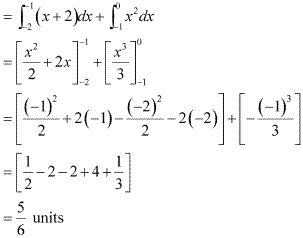11. Using the method of integration find the area bounded by the curve |x| + |y| = 1.
[Hint: The required region is bounded by lines x + y = 1, x– y = 1, – x + y = 1 and – x – y = 1].
Solution
The area bounded by the curve, |x| + |y| = 1, is represented by the shaded region ADCB as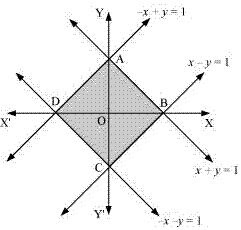The curve intersects the axes at points A(0, 1), B(1, 0), C(0, -1), and D(-1, 0).
It can be observed that the given curve is symmetrical about x - axis and y - axis.
∴Area ADCB = 4 × Area OBAO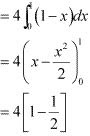= 4(1/2)
= 2 units.

12. Find the area bounded by curves {(x, y) : y ≥ x2  and y = |x|}.
Solution
The area bounded by the curves, {(x, y) : y ≥ x2 and y = |x|}, is represented by the shaded region as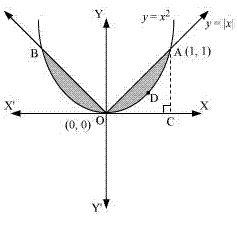It can be observed that the required area is symmetrical about y - axis.
Required area = 2[Area (OCAO) - Area (OCADO)]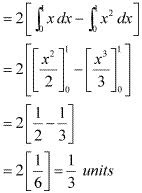13. Using the method of integration find the area of the triangle ABC, coordinates of whose vertices are A(2, 0), B (4, 5) and C (6, 3).
Solution
The Vertices of ΔABC are A(2, 0), B(4, 5) and C(6, 3).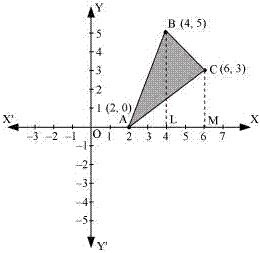Equation of line segment AB is
y - 0 = [(5 - 0)/(4 - 2)] (x - 2)
⇒ 2y = 5x - 10
⇒ y = (5/2) (x - 2) ...(1)
Equation of line segment BC is
y - 5 = [(3 - 5)(6 - 4)] (x - 4)
⇒ 2y - 10 = -2x + 8
⇒ 2y = -2x + 18
⇒ y = -x + 9 ...(2)
Equation of line segment CA is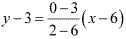⇒ -4y + 12 = -3x + 18
⇒ 4y = 3x - 6
⇒ y = (3/4)(x - 2) ...(3)
Area (ΔABC) = Area (ABLA) + Area (BLMCB) - Area(ACMA)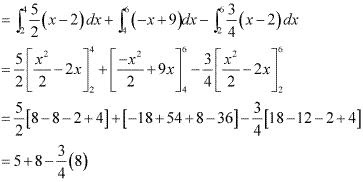= 13 - 6
= 7 units

14. Using the method of integration find the area of the region bounded by lines: 2x + y = 4, 3x – 2y = 6 and x – 3+ 5 = 0
Solution
The given equations of lines are
2x + y= 4 ...(1)
3x -2 y = 6 ...(2)
And, x - 3y + 5 = 0 ...(3)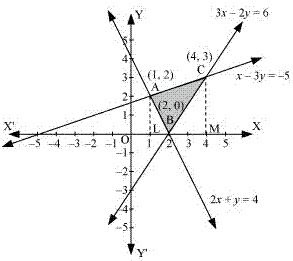The area of the region bounded by the lines is the area of ΔABC. AL and CM are the perpendiculars on x - axis.
Area (ΔABC) = Area (ALMCA) - Area (ALB) - Area(CMB)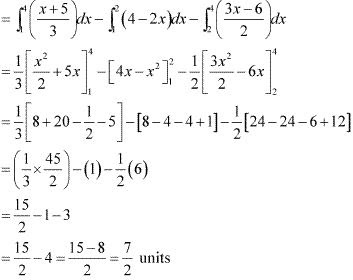15. Find the area of the region {(x, y) : y2  ≤ 4x, 4x2  + 4y2  ≤ 9}
Solution
The area bounded by the curves, {(x, y) : y2  ≤ 4x, 4x2  + 4y2  ≤ 9}, is represented as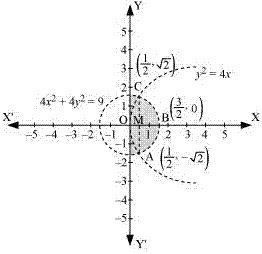The points of intersection of both the curves are (1/2, √2) and (1/2, -√2).
The required area is given by OABCO.
It can be observed that area OABCO is symmetrical about x - axis.
Area OABCO = 2 × Area OBC
Area OBCO = Area OMC + Area MBCPut 2x =  t
⇒ dx = dt/2
When x = 3/2, t = 3 and when x = 1/2, t = 1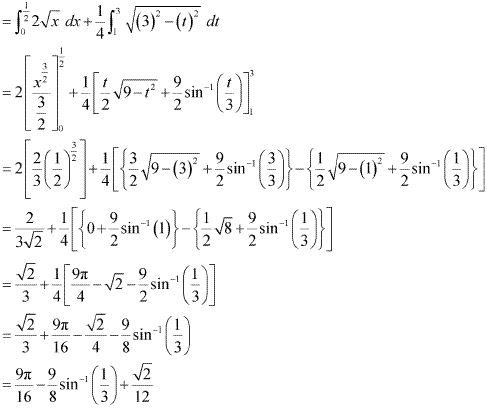Therefore, the required area is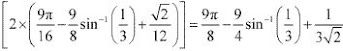units

16. Choose the correct answer Area bounded by the curve y = x3, the x-axis and the ordinates x = –2 and x = 1 is
A. – 9
B. -15/4
C. 15/4
D. 17/4
Solution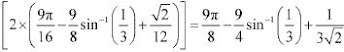Required area = A(DCOD) + A(OAB)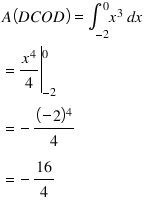Since the area is positive
∴ A(DCOD) = 16/4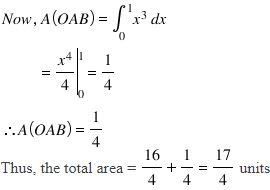Hence, the required area of the region is 17/4 units

17. Choose the correct answer The area bounded by the curve y = x | x| ,, x-axis and the ordinates x = –1 and x = 1 is given by
[Hint: y = x2 if x > 0 and y = –x2 if x < 0]
A. 0
B. 1/3
C. 2/3
D. 4/3
Solution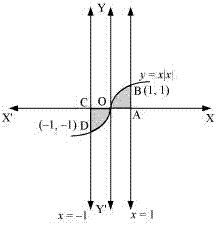Required area = A(DCOD) + A(OAB)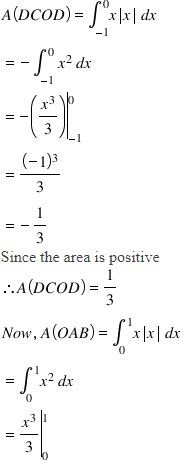= 1/3
∴ A(OAB) = 1/3
Thus, the total area = 1/3 + 1/3 = 2/3 units
Hence, the required area of the region is 2/3 units.

18. Choose the correct answer The area of the circle x2 + y2 = 16 exterior to the parabola y2 = 6x is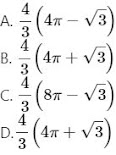Solution
The given equations are
x2 + y2 = 16 ...(1)
y2 = 6x ...(2)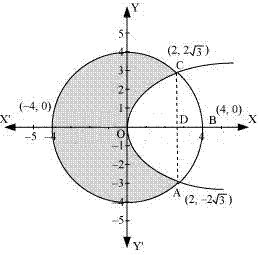Area bounded by the circle and parabola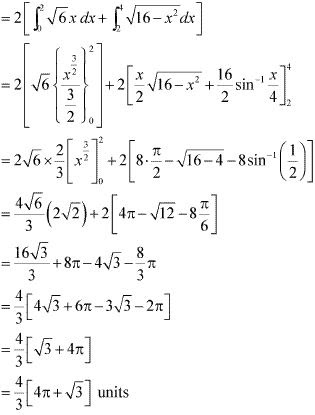Area of circle = π(r)2
= π(4)2 = 16π units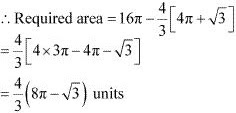Thus, the correct answer is C.

19. The area bounded by the y-axis, y = cos x and y = sin x when 0 <= x <= π2
(A) 2 ( 2 −1)
(B) √2-1
(C) √2+1
(D) √2
Solution
The given equation are
y = cos x ...(1)
And, y = sin x ...(2)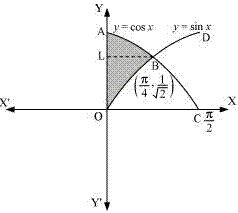Required area = Area (ABLA) + area (OBLO)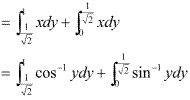Integrating by parts, we obtain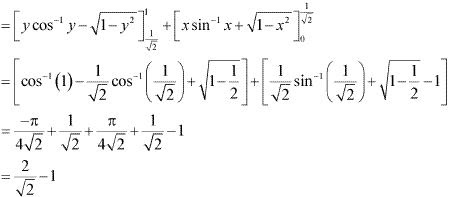= √2 - 1 units
Thus, the correct answer is B.